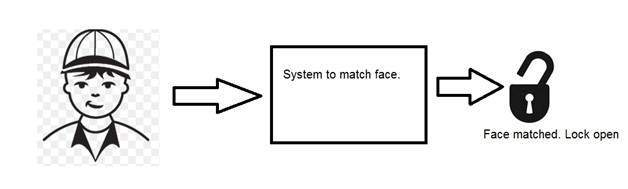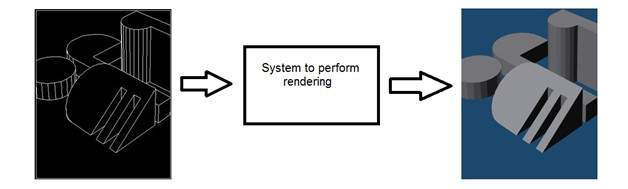# Digital Image Processing Introduction

## Introduction

Signal processing is a discipline in electrical engineering and in mathematics that deals with analysis and processing of analog and digital signals , and deals with storing , filtering , and other operations on signals. These signals include transmission signals , sound or voice signals , image signals , and other signals e.t.c.

Out of all these signals , the field that deals with the type of signals for which the input is an image and the output is also an image is done in image processing. As it name suggests, it deals with the processing on images.

It can be further divided into analog image processing and digital image processing.

## Analog image processing

Analog image processing is done on analog signals. It includes processing on two dimensional analog signals. In this type of processing, the images are manipulated by electrical means by varying the electrical signal. The common example include is the television image.

Digital image processing has dominated over analog image processing with the passage of time due its wider range of applications.

## Digital image processing

The digital image processing deals with developing a digital system that performs operations on an digital image.

## What is an Image

An image is nothing more than a two dimensional signal. It is defined by the mathematical function f(x,y) where x and y are the two co-ordinates horizontally and vertically.

The value of f(x,y) at any point is gives the pixel value at that point of an image.The above figure is an example of digital image that you are now viewing on your computer screen. But actually , this image is nothing but a two dimensional array of numbers ranging between 0 and 255.

 128 30 123 232 123 321 123 77 89 80 255 255

Each number represents the value of the function f(x,y) at any point. In this case the value 128 , 230 ,123 each represents an individual pixel value. The dimensions of the picture is actually the dimensions of this two dimensional array.

## Relationship between a digital image and a signal

If the image is a two dimensional array then what does it have to do with a signal? In order to understand that , We need to first understand what is a signal?

### Signal

In physical world, any quantity measurable through time over space or any higher dimension can be taken as a signal. A signal is a mathematical function, and it conveys some information.

A signal can be one dimensional or two dimensional or higher dimensional signal. One dimensional signal is a signal that is measured over time. The common example is a voice signal.

The two dimensional signals are those that are measured over some other physical quantities. The example of two dimensional signal is a digital image. We will look in more detail in the next tutorial of how a one dimensional or two dimensional signals and higher signals are formed and interpreted.

### Relationship

Since anything that conveys information or broadcast a message in physical world between two observers is a signal. That includes speech or (human voice) or an image as a signal. Since when we speak , our voice is converted to a sound wave/signal and transformed with respect to the time to person we are speaking to. Not only this , but the way a digital camera works, as while acquiring an image from a digital camera involves transfer of a signal from one part of the system to the other.

## How a digital image is formed

Since capturing an image from a camera is a physical process. The sunlight is used as a source of energy. A sensor array is used for the acquisition of the image. So when the sunlight falls upon the object, then the amount of light reflected by that object is sensed by the sensors, and a continuous voltage signal is generated by the amount of sensed data. In order to create a digital image , we need to convert this data into a digital form. This involves sampling and quantization. (They are discussed later on). The result of sampling and quantization results in an two dimensional array or matrix of numbers which are nothing but a digital image.

## Overlapping fields

### Machine/Computer vision

Machine vision or computer vision deals with developing a system in which the input is an image and the output is some information. For example: Developing a system that scans human face and opens any kind of lock. This system would look something like this.### Computer graphics

Computer graphics deals with the formation of images from object models, rather then the image is captured by some device. For example: Object rendering. Generating an image from an object model. Such a system would look something like this.### Artificial intelligence

Artificial intelligence is more or less the study of putting human intelligence into machines. Artificial intelligence has many applications in image processing. For example: developing computer aided diagnosis systems that help doctors in interpreting images of X-ray , MRI e.t.c and then highlighting conspicuous section to be examined by the doctor.

### Signal processing

Signal processing is an umbrella and image processing lies under it. The amount of light reflected by an object in the physical world (3d world) is pass through the lens of the camera and it becomes a 2d signal and hence result in image formation. This image is then digitized using methods of signal processing and then this digital image is manipulated in digital image processing.